# MCQs on Electrical And Electronics Measurements

##### Page 11 of 19. Go to page 1 2 3 4 5 6 7 8 9 10 11 12 13 14 15 16 17 18 19
01․ Which amplifier in used in an electronic amplifier?
Wideband amplifier.
Differential amplifier.
Power amplifier.
Butter amplifer.

Differential amplifier is a type of electronic amplifier that amplifies the difference between two voltages but does not amplify the particular voltage. For this reason, differential amplifier is used in an electronic amplifier.

02․ The circuit in figure is used to measure the power consumed by the load. The current coil and the voltage coil of the wattmeter have 0.02 Ω and 1000 Ω resistance respectively. The measured power compared to the load power will be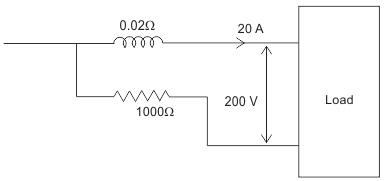0.4% less.
0.2% less.
0.2% more.
0.4 more.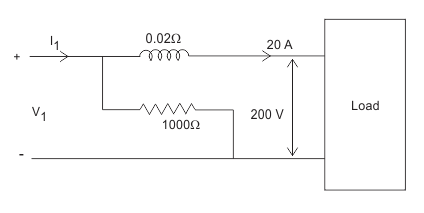Let the actual voltage and current are I1 and V1 respectively then current in CC is 20 A.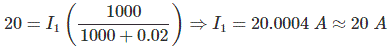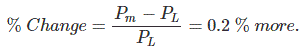V1 = 200 + 0.02 × 20 = 200.40 V. Power measured Pm = V1I1 = 200 × 200.40 = 4008 W. Load Power PL = 20 × 200 = 4000 W.

03․ A DC potentiometer is designed to measure up to about 2V with a slide wire of 800 mm. A standard cell of emf 1.18 V obtains balances at 600 mm. A test cell is seen to obtain balance at 680 mm. The emf of test cell is
1 V.
1.34 V.
1.5 V.
1.7 V.

For DC potentiometer E is proportional to the length of the wire E1/E2 = L1/L2. 1.18/E2 = 600/680. E2 = 1.34V.

04․ A metal strain gauge has a gauge factor of two. Its nominal resistance is 120 ohms. If it undergoes a strain of 10-5, the value of change of resistance in response to the strain is
0.0024 Ohm.
0.0012 Ohm.
240 Ohm.
120 Ohm.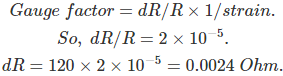05․ A wheatstone bridge cannot be used for precision measurements because errors are introduced into on account of
Contact resistance.
Thermoelectric emfs.
All of above.

Wheatstone bridge cannot be used for precision measurement because errors are introduced in contact resistance, thermoelectric EMF and resistance of connecting leads.

06․ The given figure shows wein bridge connection for frequency measurement. C and R are variables and gang together.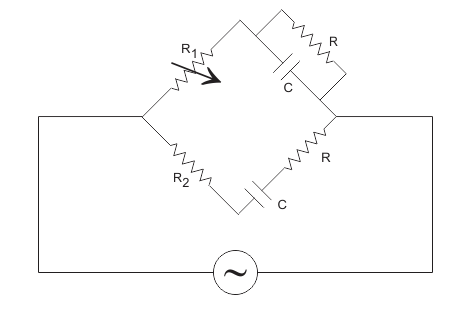For balanced condition the expression for frequency is f = 1/2πCR when
R1 = R2.
R1 = 2R2.
R1 = R2/2.
R1 = 3R2.

For balanced condition,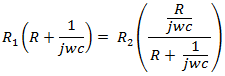Comparing the real and imaginary parts,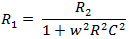As ω = 1/RC. R1 = R2/2.

07․ For the AC bridge circuit shown in figure at balance the value of Rd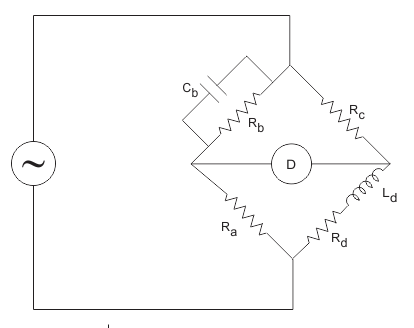will be
(Ra/Rc).Rb.
(Rc/Ra).Rb.
(Rc/Rb).Ra.
(Rb/Rc).Ra.

For balanced condition,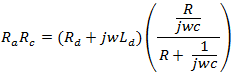Comparing the imaginary parts,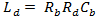After that, comparing the real parts by putting the value of Ld, Rd = (Rc/Rb).Ra.

08․ In above question no. 8, the value of Ld will be
Rb Rd Cb.
Ra Rb Cb.
Ra Rc Cb.
Rb Rc Cb.

For balanced condition,Comparing the imaginary parts,09․ When a potentiometer is used for measurement of voltage of an unknown source, the power consumed in the circuit of the unknown source under null condition is
high.
small.
ideally zero.
very high.

As a potentiometer measurement uses null or balance condition, hence no power is required for the measurement.

10․ The calibration of a voltmeter can be carried out by using
A frequency meter.
A potentiometer.
An ammeter.
A function generator.

In case of calibration of voltmeter, the main requirement is that a suitable stable DC voltage supply is available, otherwise any change in the supply voltage will cause a change in the calibration process of the the voltmeter. For this reason, a potentiometer is used for calibration of voltmeter.

<<<910111213>>>